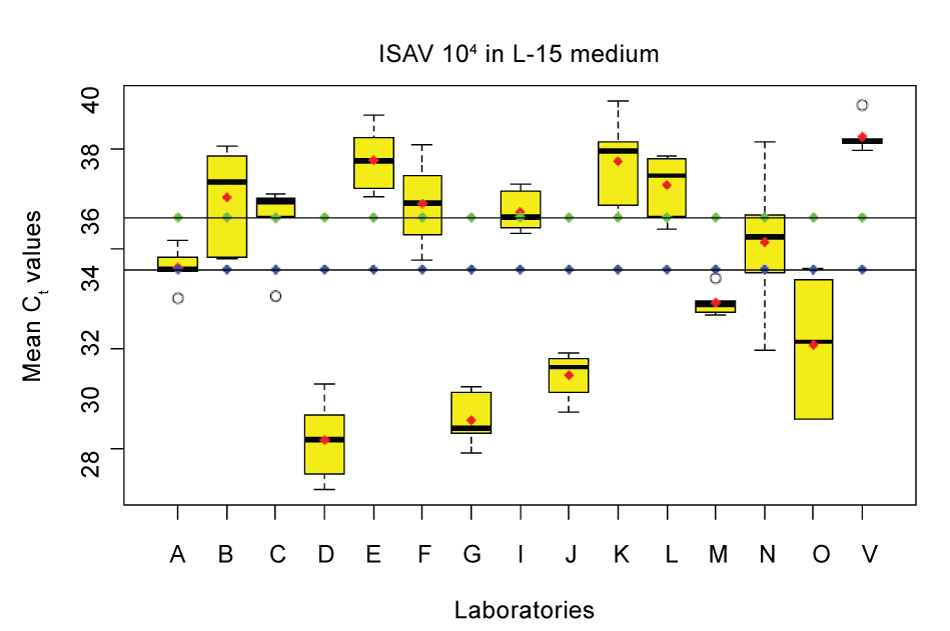Figure 4: Each box plot represents the relation to the maximum, the minimum, the mean (), the median (-) as well as the Inter-Quartile range () of the Ct values for each lab, error bars represent the lowest and the highest Ct value. Also shown are the extreme values (o). The line with (--) is the reference lab for ISA at AVC median Ct value and the line with (--) represents the overall median for all laboratories.
Goto home»